Note: This is an archvied version of our old webpage. Some links might be broken. The current one can be found here.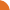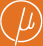Chair for Foundations of Software Reliability and Theoretical Computer Science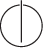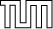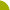Publications - Convergence of Newton's Method over Commutative SemiringsReference: M. Luttenberger and M. Schlund. Convergence of Newton's Method over Commutative Semirings. Information and Computation, ?(?):??–??, To appear. Abstract: We give a lower bound on the speed at which Newton's method (as defined in (Esparza/Kiefer/Luttenberger, 2007)) converges over arbitrary-continuous commutative semirings. From this result, we deduce that Newton's method converges within a finite number of iterations over any semiring which is ``collapsed at some'' (i.e.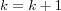holds) in the sense of (Bloom/Ésik, 2009). We apply these results to (1) obtain a generalization of Parikh's theorem, (2) compute the provenance of Datalog queries, and (3) analyze weighted pushdown systems. We further show how to compute Newton's method over any-continuous semiring by constructing a grammar unfolding w.r.t. ``tree dimension''. We review several concepts equivalent to tree dimension and prove a new relation to pathwidth. Suggested BibTeX entry: @article{LSInfComp15,     author = {M. Luttenberger and M. Schlund},     journal = {Information and Computation},     number = {?},     pages = {??--??},     title = {Convergence of {N}ewton's {M}ethod over {C}ommutative {S}emirings},     volume = {?},     year = {To appear} }